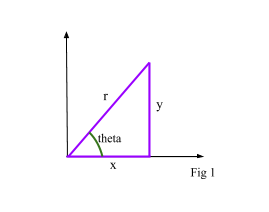# Solving Trigonometric Equations

Contents

## Summary

To solve trigonometric equations, several identities and formulas are used i.e:

•$tan\theta \quad =\quad \frac { sin\theta }{ cos\theta }$
•${ sin }^{ 2 }\theta \quad +\quad { cos }^{ 2 }\theta \quad =\quad 1$
•${ cosec }^{ 2 }\theta \quad =\quad 1\quad +\quad { cot }^{ 2 }\theta$
•${ sec }^{ 2 }\theta \quad =\quad 1\quad +\quad { tan }^{ 2 }\theta$
•$sin2x\quad =\quad 2sin(x)cos(x)$
•$cos2x\quad =\quad { 2cos }^{ 2 }x\quad -\quad 1\quad =\quad { 1\quad -\quad 2sin }^{ 2 }x\quad$

For basic trigonometric equations, we follow the following steps to solve them:
1.    Make sine, cosine or tangent the subject.
2.    Use any method including a calculator to find basic angles.
3.    Using quadrants, find all solutions in the given range.

#### Example #1

Q. Solve the equation$cos\theta \quad =\quad \frac { 1 }{ 2 }$  for the range$0\quad \le \quad \theta \quad \le \quad 2\pi$

Solution:

Using a calculator we can find that the basic angle$\theta \quad =\quad \frac { \pi }{ 3 }$

Now we know that cos is positive in the first quadrant and in the fourth quadrant hence another value of$\theta$ would be:$\theta \quad =\quad 2\pi \quad -\quad \frac { \pi }{ 3 } \quad =\quad \frac { 5\pi }{ 3 }$

Ans:$\theta \quad =\quad \frac { \pi }{ 3 } \quad \quad \quad \quad and\quad \quad \quad \theta \quad =\quad \frac { 5\pi }{ 3 }$

#### Trigonometric Identities

Refer to Fig 1.We know that:$sin\theta \quad =\quad \frac { y }{ r } \quad \quad \Rightarrow \quad 1\quad$$cos\theta \quad =\quad \frac { x }{ r } \quad \quad \Rightarrow \quad 2$$tan\theta \quad =\quad \frac { y }{ x } \quad \quad \Rightarrow \quad 3$

Dividing 1 by 2 we get:$tan\theta \quad =\quad \frac { sin\theta }{ cos\theta }$

This is called an ”Identity”.

Using pythagoras theorem:${ x }^{ 2 }\quad +\quad { y }^{ 2 }\quad =\quad { r }^{ 2 }$

Dividing the whole equation by${ r }^{ 2 }$:${ (\frac { x }{ r } ) }^{ 2 }\quad +\quad { (\frac { y }{ r } ) }^{ 2 }\quad =\quad 1$

Substituting the values:${ (cos\theta ) }^{ 2 }\quad +\quad { (sin\theta ) }^{ 2 }\quad =\quad 1$

We get another identity:${ sin }^{ 2 }\theta \quad +\quad { cos }^{ 2 }\theta \quad \equiv \quad 1$

where$\equiv$  means ”equivalent to” or ”identical to”.

These identities are very useful when solving many trigonometric equations.

#### Example #2

Q. Prove the identity$tan^{ 2 }\theta \quad -\quad { sin }^{ 2 }\theta \quad =\quad \frac { { sin }^{ 4 }\theta }{ { cos }^{ 2 }\theta }$

Solution:

Taking left hand side only:$=\quad tan^{ 2 }\theta \quad -\quad { sin }^{ 2 }\theta$$=\quad (\frac { sin\theta }{ cos\theta } )^{ 2 }\quad -\quad { sin }^{ 2 }\theta$$=\quad \frac { { sin }^{ 2 }\theta }{ { cos }^{ 2 }\theta } \quad -\quad { sin }^{ 2 }\theta$

Take${ sin }^{ 2 }\theta$  common:$=\quad { sin }^{ 2 }\theta (\frac { 1 }{ { cos }^{ 2 }\theta } \quad -\quad 1)$$=\quad { sin }^{ 2 }\theta (\frac { 1\quad -\quad { cos }^{ 2 }\theta }{ { cos }^{ 2 }\theta } )$$=\quad \frac { { sin }^{ 2 }\theta }{ { cos }^{ 2 }\theta } ({ sin }^{ 2 }\theta \quad +\quad { cos }^{ 2 }\theta \quad -\quad cos^{ 2 }\theta )$$=\quad \frac { { sin }^{ 4 }\theta }{ { cos }^{ 2 }\theta }$     Hence proven!

### Other trigonometric Formulas

Similarly, there are a few other formulas that help solve trigonometric equation:

•${ cosec }^{ 2 }\theta \quad =\quad 1\quad +\quad { cot }^{ 2 }\theta$
•${ sec }^{ 2 }\theta \quad =\quad 1\quad +\quad { tan }^{ 2 }\theta$
•$sin2x\quad =\quad 2sin(x)cos(x)$
•$cos2x\quad =\quad { 2cos }^{ 2 }x\quad -\quad 1\quad =\quad { 1\quad -\quad 2sin }^{ 2 }x\quad$